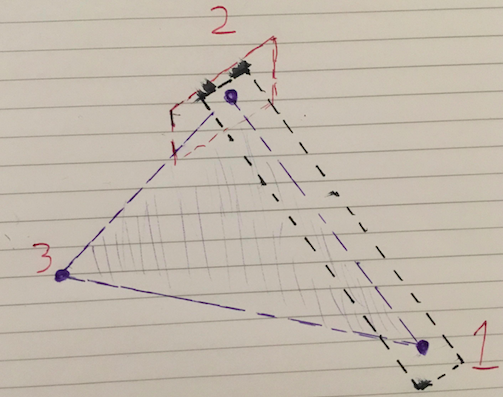# Creating planes in Unity

I am using unity and vuforia target images and I need to construct three planes. The first plane is to be constructed using three target images (consider them as points). The second plane must be perpendicular to the first and also pass through two points one and two (which are used to construct the first plan). The third plane must be perpendicular to first plane and normal to the vector which is formed by the 1st and second point.

The picture below demonstrates this. The blue triangle is the first plane set up using the target images (points) which are labelled 1,2 and 3. The black plane is the second and the red is the third.

Any help on how to achieve this through code would be greatly appreciated.It’s not quite clear what exactly you refer to by “plane”. Do you just mean an infinite mathematical plane? Or do you mean creating an actual visual Mesh? If you want a mesh it’s not quite clear how it should look like. With 3 points you will get a triangle for the first one. For the second and third it would not be clear where you want to place the 3rd point (yes, perpendicular to the first plane but that third point could be anywhere on that plane).

I’ll assume you talk about creating an infinite plane that you can raycast against. If you actually wanted to create a mesh you have to be much more clear what you want to create.

For a mathematical plane Unity has the very simple Plane struct. It has several options to create a plane. So for the first one, assuming A, B and C are your 3 corner points as Vector3, you would simply do:

``````Plane groundPlane = new Plane(A, C, B);
``````

It’s A-C-B because the points need to be ordered clockwise in order for the plane to face up.

For the other two plane all we need is the perpendicular upward direction of the first plane. For this we simply calculate the cross product between AC and AB. Note that the order matters.

``````Vector3 up = Vector3.Cross(C - A, B - A).normalized;
``````

Now you can simply construct a new point “D” by adding your newly created up vector to your point A. Finally we can construct the other two planes with the points A,B,D and A,C,D. The wanted order / orientation of those planes is not clear from your drawing. So the rest is up to you.

``````Vector3 D = A + up * wantedDistanceFromA;

Plane planeThroughAandB = new Plane(A,B,D);
Plane planeThroughAandC = new Plane(A,C,D);
``````